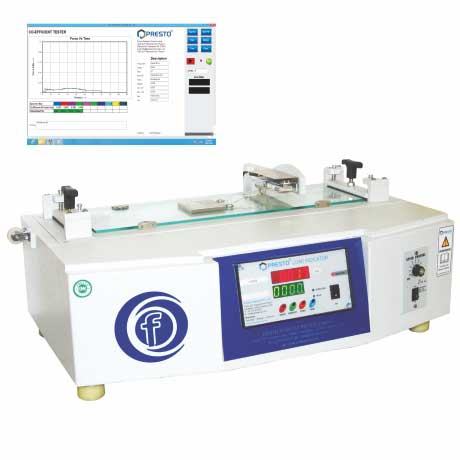# Judge The Frictional Properties Of Packaging Materials With Co-Efficient Of Friction Tester• Static Result – Static coefficient of Friction forces controls the stationery objects efficiently right from the initial stage from where it starts moving. Hence, it implies that the static Coefficient of friction is the force that controls the movement of a motionless object on a comparatively hard as well as a smooth surface. The static result of the coefficient of friction is computed by examining the starting force which is required to move the sled and by dividing the value with the weight.
• Kinetic Result – Once the static friction result of coefficient of friction is achieved, kinetic friction of the material is started. Kinetic Coefficient of friction is the strength that controls the consistent motion. The kinetic friction is determined by the force to restrict the movement of objects that glides on hard and smooth surfaces. The kinetic results can be calculated by measuring the average force that hold the movement of an object while commencing the test and divided by the load of the sled which hold another substrate on which the COF test is performed. Don’t forget that the highest force of the static result is not the part of the average force.

The Co-efficient of friction test is an essential test that must be performed to determine the frictional properties of the materials like films, labels etc. The best testing instrument to perform the COF test is Co-efficient of friction tester. The working principle of co-efficient of friction tester indicates that the level of frictional forces that a material bear easily without damaging the product which is packed inside the packaging material. The instrument is very easy to operate and offers highly precise results.